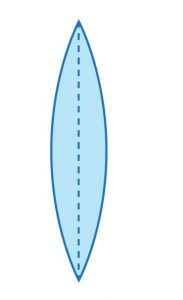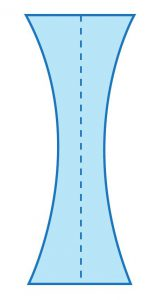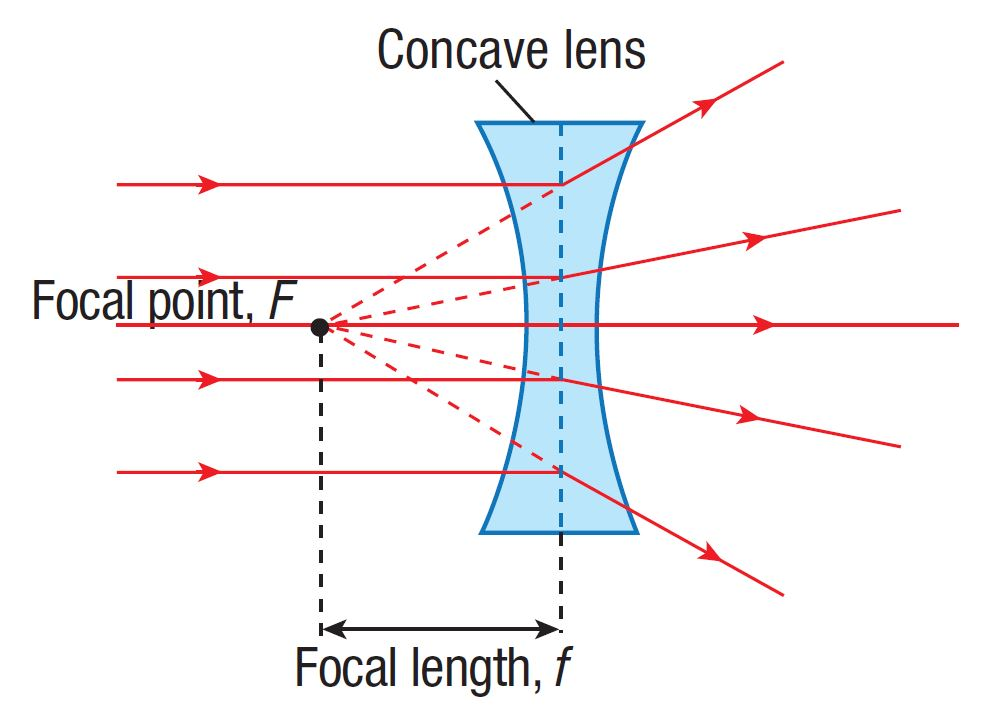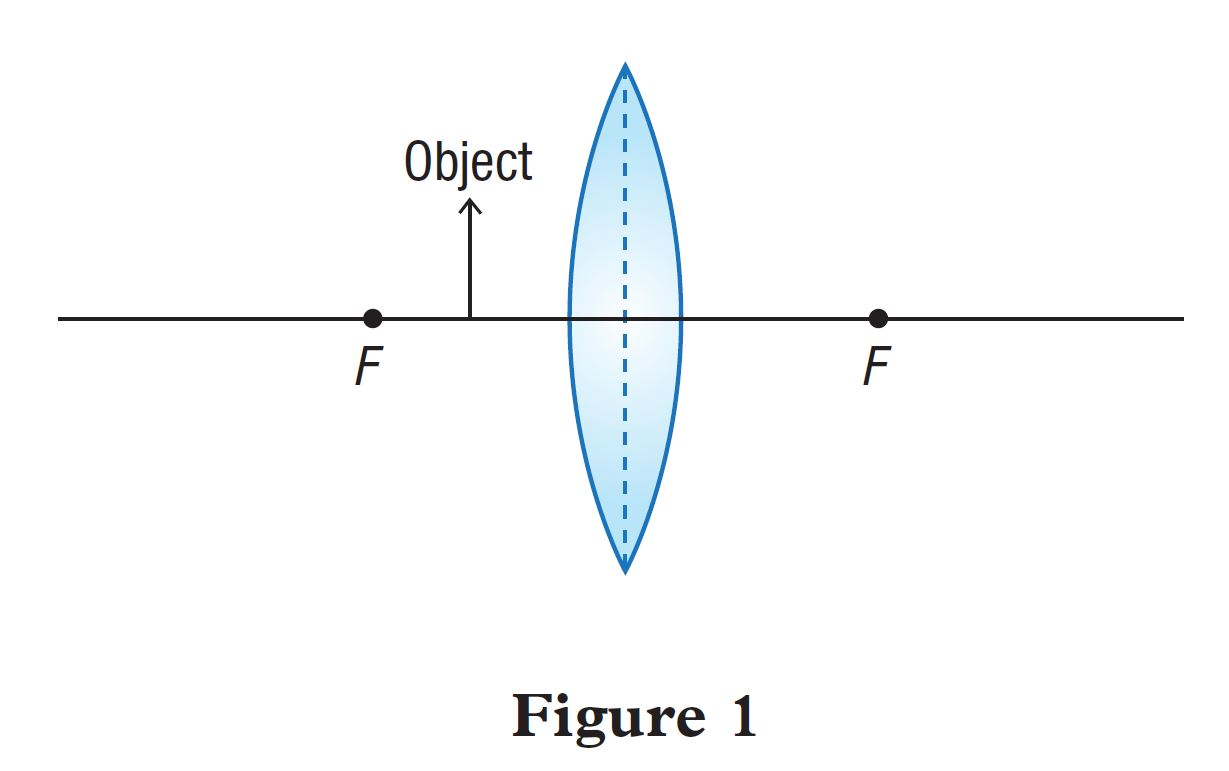# Summative Practice – Form 5 Science (KSSM) Chapter 7

Question 1:
Draw ray diagrams which describe the following characteristics of lenses:
(a) convex lens as a converging lens(b) concave lens as a diverging lens(a)(b)Question 2:
Figure 1 shows an object placed in front of a convex lens at a distance less than the focal length, ƒ.(a) Draw a ray diagram in Figure 1 to determine the image formed by the convex lens.

(b) State the characteristics of the image formed by the convex lens in Figure 1.

(a)(b) Virtual, upright, magnified

Question 3:
(a) Why do smartphones have several cameras?

(b) A student carried out an experiment to form a virtual image using a convex lens. Explain how the student formed the virtual image based on the apparatus set-up in Figure 2.Figure 2

(i) Write the problem statement.

(ii) State the position of the object (at P, Q or R).

(iii) Using an arrow (↑) as the object, draw a ray diagram to show the formation and position of the image. Complete Figure 2 to obtain your answer.

(iv) State two other characteristics of the image formed in 3(b)(iii).